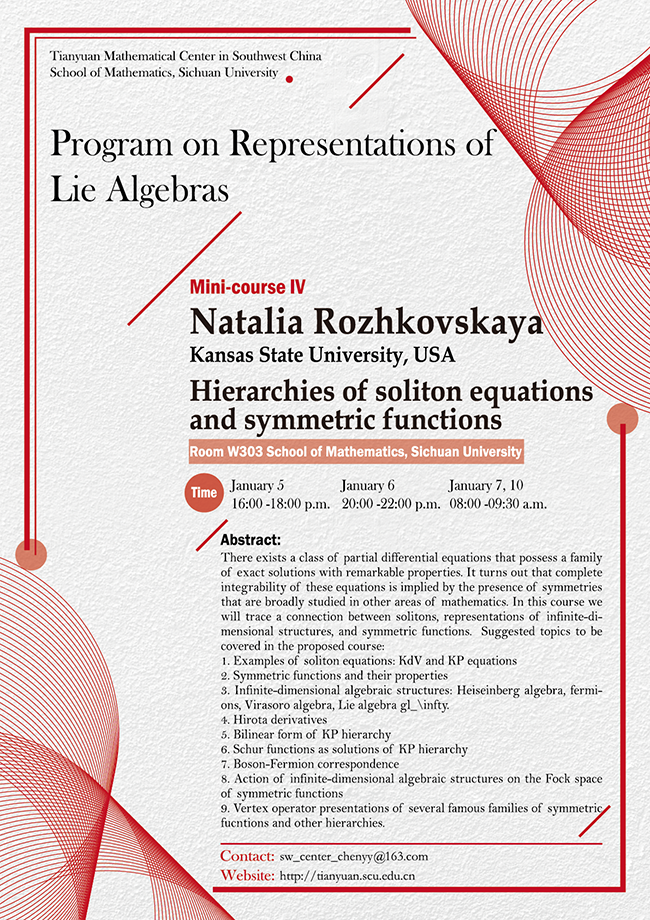2020 Thematic Program (I)

### Hierarchies of soliton equations and symmetric functions

#### W303  School of Mathematics, Sichuan University#### SPEAKER

Natalia Rozhkovskaya (Kansas State U, USA)

#### ABSTRACT

There exists a class of partial differential equations that possess a family of exact solutions with remarkable properties. It turns out that complete integrability of these equations is implied by the presence of symmetries that are broadly studied in other areas of mathematics. In this course we will trace a connection between solitons, representations of infinite-dimensional structures, and symmetric functions.  Suggested topics to be covered in the proposed course:

1. Examples of soliton equations: KdV and KP equations

2. Symmetric functions and their properties

3. Infinite-dimensional algebraic structures: Heiseinberg algebra, fermions, Virasoro algebra, Lie algebra gl_\infty.

4. Hirota derivatives

5. Bilinear form of KP hierarchy

6. Schur functions as solutions of KP hierarchy

7. Boson-Fermion correspondence

8. Action of infinite-dimensional algebraic structures on the Fock space of symmetric functions

9. Vertex operator presentations of several famous families of symmetric fucntions and other hierarchies.